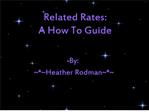# Related Rates: A How To Guide - PowerPoint PPT PresentationDownload PresentationRelated Rates: A How To Guide

Related Rates: A How To Guide
Download Presentation## Related Rates: A How To Guide

- - - - - - - - - - - - - - - - - - - - - - - - - - - E N D - - - - - - - - - - - - - - - - - - - - - - - - - - -
##### Presentation Transcript

1. Related Rates: A How To Guide By: ~*~Heather Rodman~*~

2. An Example Problem Here is an example problem that will be used and done as we go along with some general guidelines on related rates: In a conical tank, suppose the height is changing at a rate of -.2 feet per minute and the radius is changing at a rate of -.1 feet per minute. What is the rate of change in the volume when the radius is 1 foot and the height is 2 feet? Note: Problem found in Calculus with Analytic Geometry 7th edition by Ron Larson, Robert P. Hostetler, Bruce H. Edwards, and David E. Heyd on page 144. The solving of this equation will continue throughout the power point and so this reference applies to the continuation of this problem. (Larson)

3. A Good Strategy 1. Always make a sketch if they do not provide one. 99% of the time, the problems involve some sort of shape/geometry (schwartz). 2. Label the sketch using correct variables (ex. L = length, etc.) (schwartz).

4. A Good Strategy 3. Make a list of the given information and label the units and what they represent so that you do not mix up any variables (Schwartz). The home-made AP Calc book suggests making a table of values. One side for constants (variables not changing) and another for numbers that change at different given times (as in the rates or the derivatives). This way you can recognize the difference between the derivative values and the constant values (Schwartz).

5. A Good Strategy 4. Find an equation that ties all of the given information together in one way or another (Schwartz). Example: If they discuss areas, use an area equation. If they talk of volumes use a volume equation. If they use a triangle try a trig function or the Pythagorean formula. It always depends on the problem! 5. **As a check, you should always use all of the information (may be at different parts if a portfolio though) given at least once!**

6. A Good Strategy 6. Take the derivative of the equation you are using to relate all of the variables, usually in respect to time (Schwartz). It is generally easier to identify where to stick the variables after if you use the formal way of writing derivatives. For Example: vs.

7. A Good Strategy 7. When you take the derivative of something such as variable r, do not forget to multiply it by its derivative, such as !

8. A Good Strategy 8. Plug in all of the variables. You should end up with one variable to find, usually ending up as your final answer (Schwartz). 9. Note: If you end up with more than one variable, you will need to use some sort of equation that goes along with the problem given to solve for one of the variables you need (Schwartz).

9. A Good Strategy 10. Finally, do not forget labels! Label your answer with correct units (99% of the time you will have to do this) and make sure that this is the answer they are looking for (Schwartz).

10. Special Tip If given the information to use two variables, set up a ratio and solve for one so that taking the derivative is easier for you. Note that numerical values must be assigned to the constants (non-derivatives) of the variables for a ratio to be used.

11. Another Example Problem An oil tank spills oil that spreads in a circular pattern whose radius increases at the rate of 50 feet/minute. How fast is the area of the spill increasing when the radius of the spill is 20 feet? Problem found in the AB Calculus Manual by Stu Schwartz on the Master Math Mentor website. Note: the problem is edited to make it simpler and with less parts to it.

12. Example

13. Example

14. Works Cited Larson, Ron, et al. Calculus with Analytic Geometry. Boston: Houghton, 2002. Schwartz, Stu. AB Calculus Manual. Master Math Mentor. 21 May 2007 <http://www.mastermathmentor.com/mmm/calc/def ault.aspx?page=ABManual>.